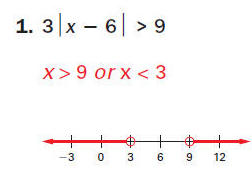# One-step inequalities

One-step inequalities are very easy to solve. They are very similar to one-step equations when it comes to the solving procedure. The biggest difference is that, while the solution of an equation is one number, the solution of an inequality is often a large group of numbers. For instance, let us say you have to solve an inequality that looks like this:

x + 4 > 8

The first thing  you have to do is to move the number 4 to the right side of the inequality. Do not forget to change the sign of the number you are moving to the other side. After that, just perform the subtraction. Like this:

x > 8 – 4

x > 4

As you can see, the inequation states that x is greater than 4. That means that the solution of the inequation are all numbers greater than 4.Also, a very important thing to remember is to change the sign of inequality when multiplying or dividing the whole expression with a negative number. For example, let us say we have an expression that looks like this:

-4x >16

To get to the value of x, we have to divide the whole expression with (-4). Like this:

-4x > 16 |: (-4)

Now we have to change that sign, or else we will get the wrong result. The final result will be:

x < -4

You can check that result by inserting any value smaller than (-4) as the value of x. If you wish to practice solving one-step inequalities, feel free to use the free math worksheets below.